Setup for 3 Player a Game of Food Chain Magnate

Originally generated on 10/9/2018 5:45:16 PM

Player Setup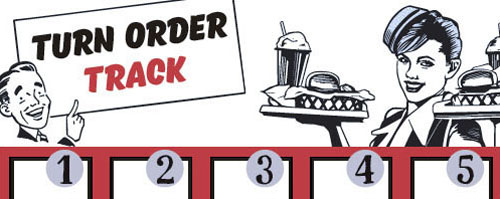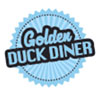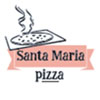Other Setup

Bank starts with \$150.00
Remove these Billboards: #15, #16
Number of 1x Employee cards used = 1

Map (Dehydration Land)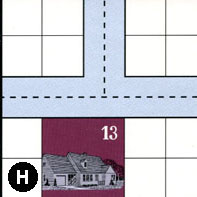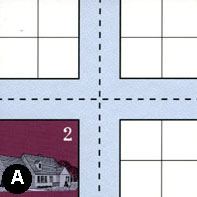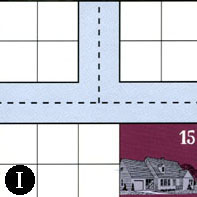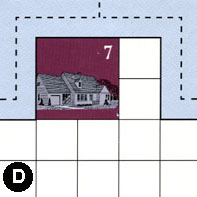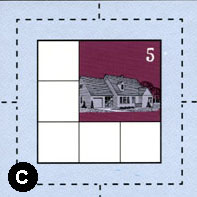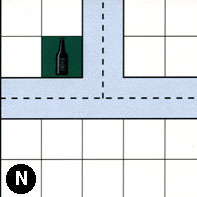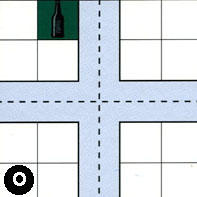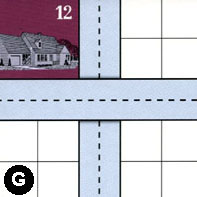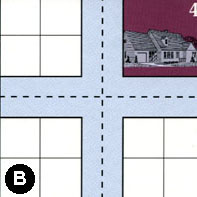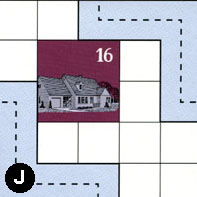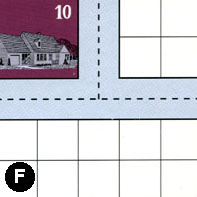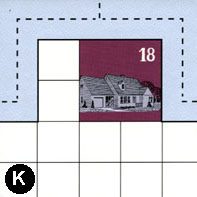Map Stats

Map Option = Dehydration Land
Total Number of Tiles = 12
Total Number of Starting Houses = 10
Total Number of Beer Spots = 2
Total Number of Soda Spots = 0
Total Number of Lemonade Spots = 0
Total Number of Drink Spots = 2
Number of independent path systems = 2
Number of independent neighborhoods = 14

Path System 1
Size = Large
Number of Tiles = 12
Number of Paths = 45
Contains Loop(s) = No
Number of Starting Houses = 11
Starting Houses Pct of Map (%) = 110
Number of Beer Spots = 2
Number of Soda Spots = 0
Number of Lemonade Spots = 0
Number of Drink Spots = 2
Drink Spots Pct of Map (%) = 100
Tile Ids = A, B, C, D, F, G, H, I, J, K, N, O
Paths = HWE, CWN, CNE, BWS, JWS, NWE, AWN, IWE, DWE, DWN, GWE, KWN, KNE, KWE, DNE, IWN, OWN, NWN, ANE, AWE, INE, ONE, GNS, OWE, NNE, OWS, FWN, FNE, FWE, ONS, OES, AWS, ANS, AES, BWN, BNE, BWE, BES, CWE, HWN, HNE, CWS, CNS, CES, BNS What do these mean?
Starting House Ids = 0, 2, 4, 5, 7, 10, 12, 13, 15, 16, 18
Path System 2
Size = Tiny
Number of Tiles = 1
Number of Paths = 1
Contains Loop(s) = No
Number of Starting Houses = 1
Starting Houses Pct of Map (%) = 10
Number of Beer Spots = 0
Number of Soda Spots = 0
Number of Lemonade Spots = 0
Number of Drink Spots = 0
Drink Spots Pct of Map (%) = 0
Tile Ids = J
Paths = JEN What do these mean?
Starting House Ids = 16

Neighborhood 1
Total Size = Tiny
Number of Total Spaces = 4
Number of Empty Spaces (for new houses & gardens) = 4
Number of Beer Spots = 0
Number of Soda Spots = 0
Number of Lemonade Spots = 0
Number of Drink Spots = 0
Number of Starting Houses = 0
Starting Houses Pct of Map (%) = 0
Tile Ids = H
Neighborhood 2
Total Size = Medium
Number of Total Spaces = 28
Number of Empty Spaces (for new houses & gardens) = 24
Number of Beer Spots = 0
Number of Soda Spots = 0
Number of Lemonade Spots = 0
Number of Drink Spots = 0
Number of Starting Houses = 1
Starting Houses Pct of Map (%) = 10
Tile Ids = A, H, N
Starting House Ids = 13
Neighborhood 3
Total Size = Medium
Number of Total Spaces = 30
Number of Empty Spaces (for new houses & gardens) = 18
Number of Beer Spots = 0
Number of Soda Spots = 0
Number of Lemonade Spots = 0
Number of Drink Spots = 0
Number of Starting Houses = 3
Starting Houses Pct of Map (%) = 30
Tile Ids = A, D, I
Starting House Ids = 2, 7, 15
Neighborhood 4
Total Size = Tiny
Number of Total Spaces = 4
Number of Empty Spaces (for new houses & gardens) = 4
Number of Beer Spots = 0
Number of Soda Spots = 0
Number of Lemonade Spots = 0
Number of Drink Spots = 0
Number of Starting Houses = 0
Starting Houses Pct of Map (%) = 0
Tile Ids = H
Neighborhood 5
Total Size = Medium
Number of Total Spaces = 16
Number of Empty Spaces (for new houses & gardens) = 14
Number of Beer Spots = 2
Number of Soda Spots = 0
Number of Lemonade Spots = 0
Number of Drink Spots = 2
Number of Starting Houses = 0
Starting Houses Pct of Map (%) = 0
Tile Ids = A, I, N, O
Neighborhood 6
Total Size = Medium
Number of Total Spaces = 12
Number of Empty Spaces (for new houses & gardens) = 12
Number of Beer Spots = 0
Number of Soda Spots = 0
Number of Lemonade Spots = 0
Number of Drink Spots = 0
Number of Starting Houses = 0
Starting Houses Pct of Map (%) = 0
Tile Ids = G, I, O
Neighborhood 7
Total Size = Tiny
Number of Total Spaces = 4
Number of Empty Spaces (for new houses & gardens) = 4
Number of Beer Spots = 0
Number of Soda Spots = 0
Number of Lemonade Spots = 0
Number of Drink Spots = 0
Number of Starting Houses = 0
Starting Houses Pct of Map (%) = 0
Tile Ids = G
Neighborhood 8
Total Size = Medium
Number of Total Spaces = 9
Number of Empty Spaces (for new houses & gardens) = 5
Number of Beer Spots = 0
Number of Soda Spots = 0
Number of Lemonade Spots = 0
Number of Drink Spots = 0
Number of Starting Houses = 1
Starting Houses Pct of Map (%) = 10
Tile Ids = C
Starting House Ids = 5
Neighborhood 9
Total Size = Large
Number of Total Spaces = 37
Number of Empty Spaces (for new houses & gardens) = 33
Number of Beer Spots = 0
Number of Soda Spots = 0
Number of Lemonade Spots = 0
Number of Drink Spots = 0
Number of Starting Houses = 1
Starting Houses Pct of Map (%) = 10
Tile Ids = B, F, J, N, O
Starting House Ids = 16
Neighborhood 10
Total Size = Medium
Number of Total Spaces = 32
Number of Empty Spaces (for new houses & gardens) = 20
Number of Beer Spots = 0
Number of Soda Spots = 0
Number of Lemonade Spots = 0
Number of Drink Spots = 0
Number of Starting Houses = 3
Starting Houses Pct of Map (%) = 30
Tile Ids = F, G, K, O
Starting House Ids = 10, 12, 18
Neighborhood 11
Total Size = Tiny
Number of Total Spaces = 4
Number of Empty Spaces (for new houses & gardens) = 4
Number of Beer Spots = 0
Number of Soda Spots = 0
Number of Lemonade Spots = 0
Number of Drink Spots = 0
Number of Starting Houses = 0
Starting Houses Pct of Map (%) = 0
Tile Ids = G
Neighborhood 12
Total Size = Tiny
Number of Total Spaces = 4
Number of Empty Spaces (for new houses & gardens) = 4
Number of Beer Spots = 0
Number of Soda Spots = 0
Number of Lemonade Spots = 0
Number of Drink Spots = 0
Number of Starting Houses = 0
Starting Houses Pct of Map (%) = 0
Tile Ids = B
Neighborhood 13
Total Size = Medium
Number of Total Spaces = 32
Number of Empty Spaces (for new houses & gardens) = 28
Number of Beer Spots = 0
Number of Soda Spots = 0
Number of Lemonade Spots = 0
Number of Drink Spots = 0
Number of Starting Houses = 1
Starting Houses Pct of Map (%) = 10
Tile Ids = A, B, H, N
Starting House Ids = 13
Neighborhood 14
Total Size = Tiny
Number of Total Spaces = 4
Number of Empty Spaces (for new houses & gardens) = 0
Number of Beer Spots = 0
Number of Soda Spots = 0
Number of Lemonade Spots = 0
Number of Drink Spots = 0
Number of Starting Houses = 1
Starting Houses Pct of Map (%) = 10
Tile Ids = B
Starting House Ids = 4

v2.1.5